# Kerala Syllabus 7th Standard Maths Solutions Chapter 5 Area of a Triangle

You can Download Area of a Triangle Questions and Answers, Activity, Notes, Kerala Syllabus 7th Standard Maths Solutions Chapter 5 to help you revise the complete Syllabus and score more marks in your examinations.

## Kerala State Syllabus 7th Standard Maths Solutions Chapter 5 Area of a Triangle

### Area of a Triangle Text Book Questions and Answers

Dot Math Textbook Page No. 68

In the picture below, the horizontal and vertical dots are one centimetre apart.What are the areas of the coloured figures below?In the above picture, draw other figures by joining dots and find out their areas.
Figure 1 is 13 cm,
Figure 2 is 6 cm
Figure 3 is 12 cm.

Explanation:
The area of figure 1 is 7+6 =13 cm,
figure 2 is 2+2+2 = 6 cm,
figure 3 is 3+3+3+3 = 12 cm.

This can be done using the Grid option in GeoGebra. Select the Polygon tool and click at various intersections of the grid lines to draw different figures.Calculate the area of these. To check the answers, select the Area tool and click within each figure.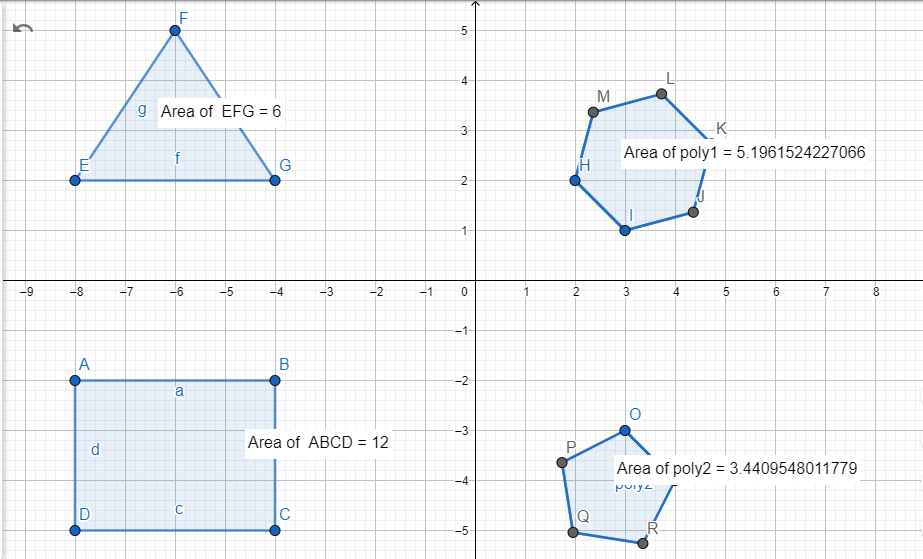Different halves
A rectangle can be halved by cutting vertically or horizontally along the middle: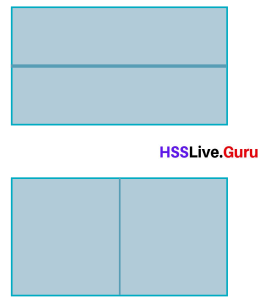We can also cut corner to corner to make two triangles of half the area.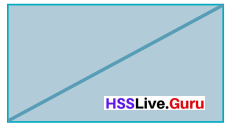What happens when we draw a slanted line through the middle’?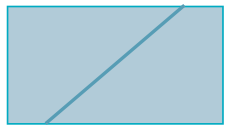Explanation:
By drawing a slanted line through the middle of the rectangle we will get two quadrilaterals.

Didn’t we get two quadrilaterals of half the area?
Such a quadrilateral with only one pair of parallel sides is called a trapezium.Parallelogram and rectangle Textbook Page No. 70

How do we compute the area of this parallelogram?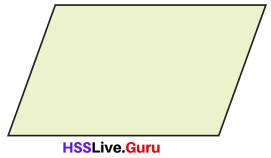We can compute the area of this parallelogram by multiplying the base of the perpendicular by its height.

Cut out a right angled triangle from the left as shown below.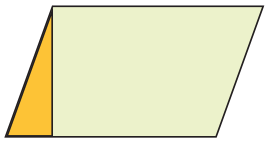And place it on the right like this: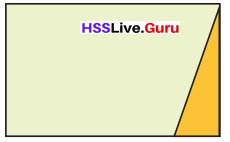Now we get a rectangle of the same area.

Rectangle and triangle
In this picture below, what part of the rectangle is the red triangle?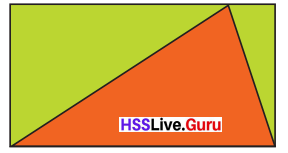Here, the red triangle is half part of the rectangle as the rectangle surrounds the triangle.

Rectangle and triangle Textbook Page No. 72
How about cutting the rectangle into two smaller rectangles?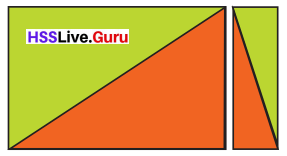The area of the red triangle in each of these pieces is half the area of that piece. So the sum of their areas is equal to half the area of the original rectangle.
The original red triangle is made up of these two smaller red triangles.
Thus the area of the original red triangle is half the area of the original rectangle.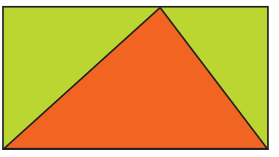What about the red triangle above?Explanation:
When the red triangle in the image above is divided in two, a square and a rectangle are formed.
The square and the rectangle are made up of these two smaller red triangles.
The area of square and rectangle will be the sum of two formed half triangles. The area of original triangle will be the half the area of square and rectangle.

Draw a rectangle in GeoGebra and mark a point on the top side. Select the Polygon tool and draw a triangle as in the problem. Colour it red. Select the Area tool and find the area of the triangle.
Drag the point on top and see what happens to the area.

Here, we have drawn a rectangle in GeoGebra and marked a point on the top side and selected the Polygon tool and drawn a triangle, and selected the area tool.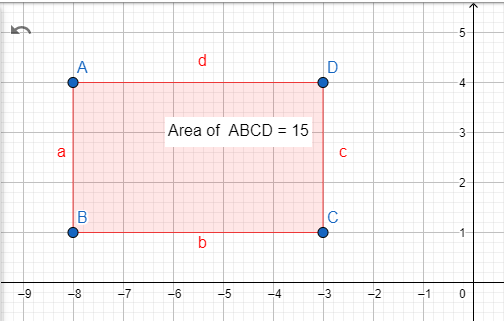Rectangles within rectangle
Look at this rectangle:Do you see any relation between the areas of the red rectangles?
Think for a moment before turning the page for the answer.Rectangles within rectangle Textbook Page No. 74
The diagonal of the large rectangle divides it into two right angled triangles of equal area. Each of these triangles is made up of the red rectangle within it and two small right angled triangles.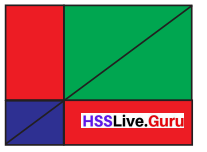The areas of right angled triangles of the same colour are equal.
So, the area of the red rectangles are also equal.
What if we draw rectangle through some other point on the diagonal?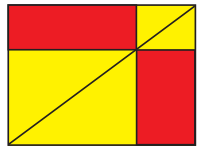The areas of right angled triangles with the same colour are equal even if we draw a rectangle via another point on the diagonal.
So, the area of the red rectangles will also be equal.

In GeoGebra, draw a pair of horizontal lines, 8 units apart. On the bottom line, mark two points D and F 4 units apart. Mark a point B on the top line and draw ΔDFB using the Polygon tool. What is the area of this triangle? Check the answer using the Area tool. Now drag B and see what happens to the area.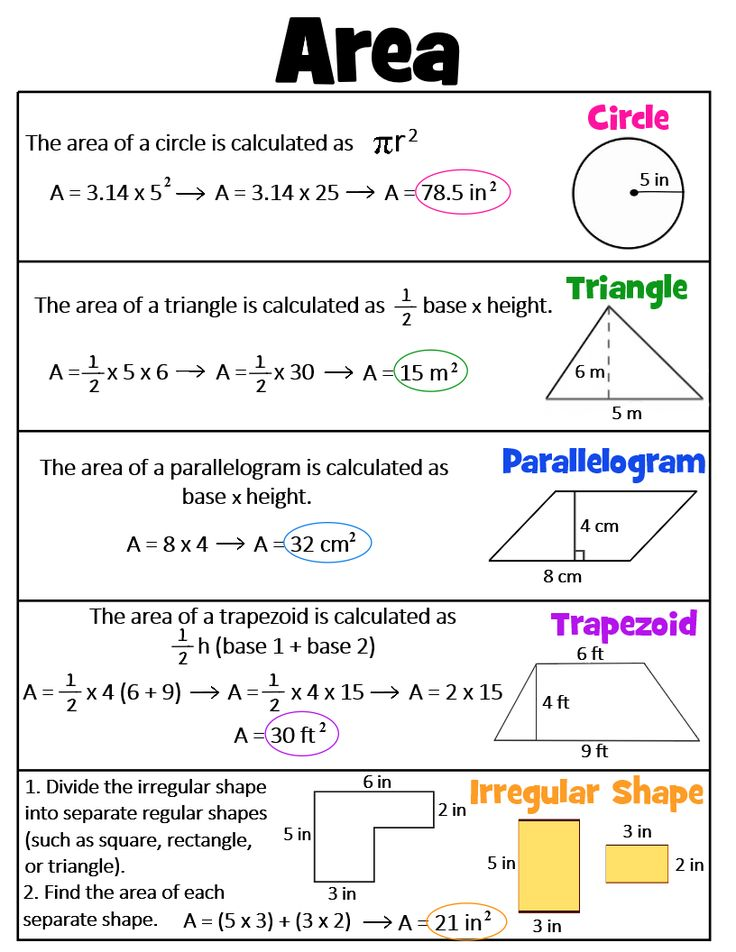Square division
Draw a square and mark the mid points of its sides.Join these to the corners of the square as shown below: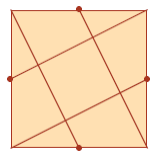We get a square at the centre: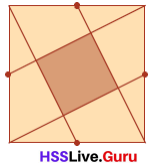What part of the original square is this?

Square division Textbook Page No. 76

Cut out a figure like this in paper.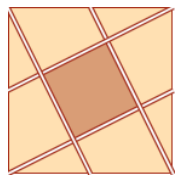Rearrange the triangular pieces like this: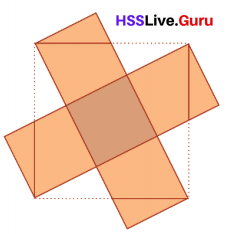Now we get five squares of equal size.
So, the small square is $$\frac{1}{5}$$ of the orignal square.Trapezium Textbook Page No. 77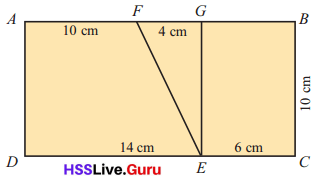In the figure ABCD is a rectangle and EFG is a right angled triangle.
What are the areas of the trapeziums AFED, and ECBF?

Explanation:
Given that the figure ABCD is a rectangle and EFG is a right-angled triangle. So the areas of the trapeziums AFED, and ECBF is
Here, the total area will be
= 20 × 10
= 200 sq cm.
The area of the rectangle is ECBG
= EC × BC
= 6 × 10
= 60 sq cm.
The area of the triangle EGF is
= $$\frac{1}{2}$$ × 4 × 10
= 2 × 10
= 20 sq cm.
So the area of the trapezium ECBF is
= area of the rectangle is ECBG + area of the triangle EGF
= 60 + 20
= 80 sq cm.

Halving Textbook Page No. 68

Cut out a paper rectangle 4 centimetres wide and 3 centimetres high.Draw a line down the middle as below:Now we have two rectangles. What is the area of each?
To see that it’s half the big rectangle, we need only fold it across.
So the area of a small rectangle is half the area of the large rectangle. That is,
$$\frac{1}{2}$$ × 12 = 6 square centimetres
Can you halve the area in any other way?The image above shows another method for halving the area.
The diagonal of the rectangle divides it into two triangles of equal area.
Area of triangle will be $$\frac{1}{2}$$×12 = 6 square centimetres.
Therefore, the area of original rectangle will be twice of the triangle , 2×6 = 12 square centimetres.Another half
Cut out a paper rectangle 10 centimetres wide and 8 centimetres high.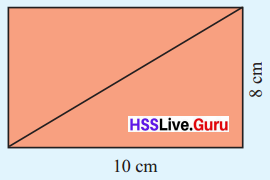Draw a line from corner to corner as shown.
The rectangle is split into two triangles.
Are their areas equal?
Can we fold and check as before?
Then put one on top of the other.
So, what is the area of each triangle?
The area of a triangle is half the area of the rectangle. That is,
$$\frac{1}{2}$$ × 10 × 8 = 40 sq. cm.
Do you notice anything about the angles of these triangles?A triangle with a right angle at one corner is called a right-angled triangle.
What is the area of the right-angled triangle shown on the right?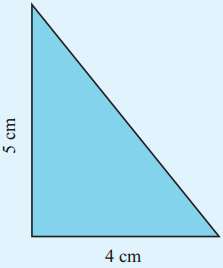The area of the right-angled triangle is 10 sq cm.

Explanation:
The area of the right-angled triangle is
= $$\frac{1}{2}$$ × 4 × 5
= 2 × 5
= 10 sq cm.

Cut out two right angled triangles like this and place them together as shown below.What is the area of this rectangle?
The area of the right-angled triangle is half of this, right?
Area of the triangle = $$\frac{1}{2}$$ × 4 × 5
= 10 sq. cm.
In this, 4 and 5 are the lengths of the perpendicular sides of the right-angled triangle.
Thus we have the method to compute the area of a right-angled triangle.

The area of a right-angled triangle is half the product of the perpendicular sides.

Now calculate the area of the figures shown below:The area of the figures is 30 cm sq, 8.75 cm sq, 13.5 cm sq.

Explanation:
The area of the first figure is
= $$\frac{1}{2}$$ × 10 × 6
= 5 × 6
= 30 cm sq.
The area of the second figure is
= $$\frac{1}{2}$$ × 3.5 × 5
= $$\frac{17.5}{2}$$
= 8.75 cm sq.
The area of the third figure is
= $$\frac{1}{2}$$ × 4.5 × 6
= 4.5 × 3
= 13.5 cm sq.The total area is 40 cm sq.

Explanation:
The area of the rectangle is length × breadth
= 6 × 5
= 30 cm sq.
And the area of the triangle is
= $$\frac{1}{2}$$ × 2 × 5
= 5 cm sq.
The total area is the area of the rectangle + the area of the triangle
= 30 + 5 + 5
= 40 cm sq.

The total area is 16.5 cm sq.

Explanation:
The area of the rectangle is length × breadth
= 4 × 3
= 12 cm sq.
The area of the first triangle is
= $$\frac{1}{2}$$ × 1 × 3
= $$\frac{3}{2}$$
= 1.5 cm sq.
The area of the second triangle is
= $$\frac{1}{2}$$ × 2 × 3
= 3 cm sq.
The total area is the area of the rectangle + the area of the triangle
= 12 + 1.5 + 3
=16.5 cm sq.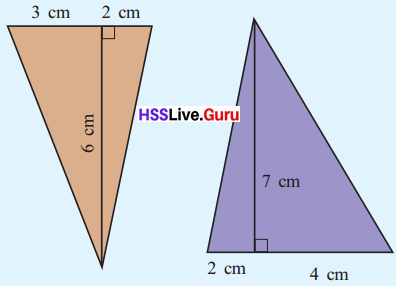The total area of the triangle is 15 cm sq.

Explanation:
The area of the first triangle is
= $$\frac{1}{2}$$ × 3 × 6
= 3 × 3
= 9 cm sq.
The area of the second triangle is
= $$\frac{1}{2}$$ × 2 × 6
= 6 cm sq.
The total area of the triangle is
= 9 + 6
=15 cm sq.

• The area of a right-angled triangle is 96 square centimeters. One of the perpendicular sides is 16 centimeters long. What is the length of the other?

The length of the other side is 6 sq cm.

Explanation:
Given that the area of a right-angled triangle is 96 square centimeters and one side of the perpendicular sides is 16 centimeters. So the length of the other side is
96 = 16 × L
L = 96 ÷ 16
= 6 sq cm.

• The perpendicular sides of a right-angled triangle are 12 and 15 centimeters long. Another right angled triangle of the same area has one of the perpendicular sides 18 centimeters long. What is the length of the other?

The area of the first right-angled triangle is 90 sq cm and the length of the other side is 10 cm.

Explanation:
Given the perpendicular sides of a right-angled triangle are 12 and 15 centimeters long. So the area of the first triangle is
= $$\frac{1}{2}$$ × 12 × 15
= 90 sq cm.
So the area will be
90 = $$\frac{1}{2}$$ × 18 × h
90 = 9 × h
h = $$\frac{90}{9}$$
= 10 cm.Other triangles Textbook Page No. 72

Look at this triangle: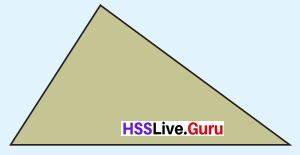No angle of it is right.
How do we calculate the area?
Can we cut it into two right-angled triangles?
Look at the earlier problems you have done.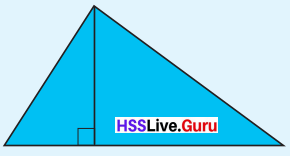So to compute the area, what all lengths are to be measured?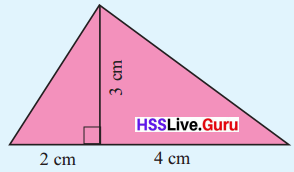Area = ($$\frac{1}{2}$$ × 2 × 3) + ($$\frac{1}{2}$$ × 4 × 3)
= 3 + 6
= 9 sq. cm.
We can calculate the area of any triangle like this.
What is the general method to calculate the area of a triangle?
Look at this triangle: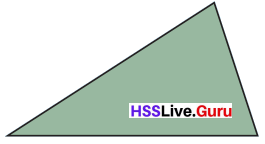To find the area, first, draw a perpendicular from the top comer to divide it into two right-angled triangles.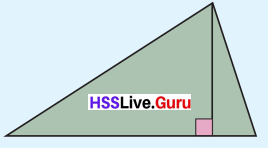Now some lengths are to be measured.
Let’s write letters for these for the time being.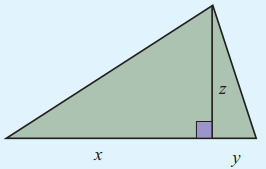So, how do we write the area?
The sum of the areas of two right-angled triangles is
($$\frac{1}{2}$$ × x × z) + ($$\frac{1}{2}$$ × y × z)
= $$\frac{1}{2}$$xz + $$\frac{1}{2}$$yz
= $$\frac{1}{2}$$(x + y)z
In this, x + y is the length of the bottom side.So, how do we compute the area of a triangle?
The area of a triangle is half the product of one side with the perpendicular from the opposite side.

Now compute the area of these figures:The area of the first triangle is 25 sq cm.
The area of the second triangle is 11 sq cm.

Explanation:
The area of the first triangle is
= $$\frac{1}{2}$$ × 5 × 10
= 5 × 5
= 25 sq cm.
The area of the second triangle is
= $$\frac{1}{2}$$ × 5.5 × 4
= 5.5 × 2
= 11 sq cm.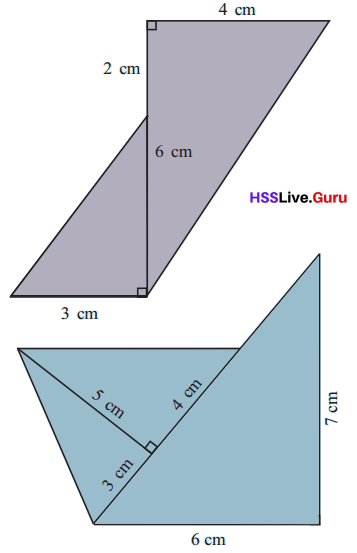The area of the first figure is 9 sq cm.
The area of the second figure is 16 sq cm.

Explanation:
The area of the first figure is
= $$\frac{1}{2}$$ × 3 × 6
= 3 × 3
= 9 sq cm.
The height of the second triangle is
= 2+6
= 8 cm and the base is 4 cm.
The area of the second figure is
= $$\frac{1}{2}$$ × 8 × 4
= 4 × 4
= 16 sq cm.

The area of the first figure is 9 sq cm.
The area of the second figure is 16 sq cm.
The area of the third figure is 21 sq cm.

Explanation:
The area of the first figure is
= $$\frac{1}{2}$$ × 3 × 5
= $$\frac{15}{2}$$
= 7.5 sq cm.
The area of the second figure is
= $$\frac{1}{2}$$ × 4 × 5
= 2 × 5
= 10 sq cm.
The area of the third figure is
= $$\frac{1}{2}$$ × 6 × 7
= 3 × 7
= 21 sq cm.
So the total area of the figure is 7.5+10+21 = 38.5 sq cm.The area of the first figure is 24 sq cm.
The area of the second figure is 24 sq cm.
The area of the rectangle is 120 sq cm.
The total area is 168 sq cm.

Explanation:
The area of the first figure is
= $$\frac{1}{2}$$ × 4 × 12
= 2 × 12
= 24 sq cm.
The area of the second figure is
= $$\frac{1}{2}$$ × 4 × 12
= 2 × 12
= 24 sq cm.
The area of the rectangle is
= l × b
= 10 × 12
= 120 sq cm.
So the total area is
= 24 + 24 + 120
= 168 sq cm.Another triangle Textbook Page No. 75
Look at this triangle: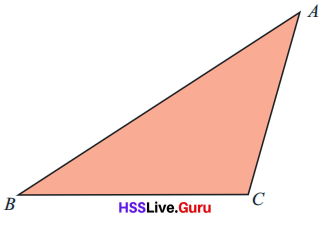How do we compute its area?
How do we draw a perpendicular from A to BC?
How about extending BC to the right’?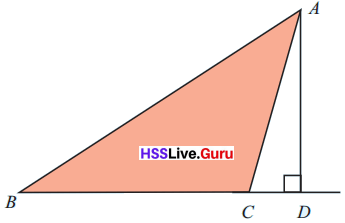Now how do we calculate the area of ΔABC?
We get ΔABC by removing ΔACD from ΔABD.

ΔABD is a right-angled triangle.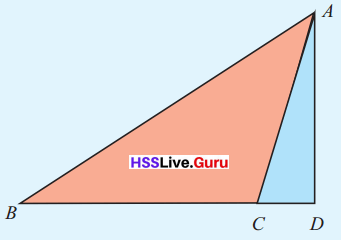Area of ΔABD = $$\frac{1}{2}$$ × BD × AD
ΔACD also is a right-angled triangle.
Area of ΔACD = $$\frac{1}{2}$$ × CD × AD
Now we can find the area of ΔABC
Area of ΔABC = Area of ΔABD – Area of ΔACD
= $$\frac{1}{2}$$ × BD × AD – $$\frac{1}{2}$$ × CD × AD
= $$\frac{1}{2}$$ × (BD – CD) × AD
From the picture,
BD – CD = BC
Thus we have
Area of ΔABC = $$\frac{1}{2}$$ × (BD – CD) × AD
= $$\frac{1}{2}$$ × BC × AD
Measure BC, and AD and compute the area.
Here AD is the height measured from BC.
So for triangles of this kind also, the area is half the product of a side and the height from it.

Look at this triangle: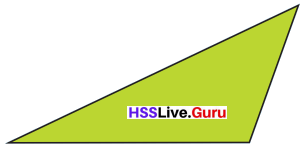Compute the area of the triangle by measuring out the needed lengths.
Let the base of triangle be 6cm wide and the height be 4cm long.
Thus, the area of triangle will be $$\frac{1}{2}$$ × 6 × 4 = 12 square cm.Let’s do it! Textbook Page No. 77

Question 1.
A rectangular plot is 30 metres long and 10 metres wide. Within it, a triangular part is marked for plant¬ing plantain.(i). What is the area of this part?
Area = 300 m sq.

Explanation:
Given that a rectangular plot is 30 meters long and 10 meters wide. So the area of the land will be 30×10 = 300 m sq.
The area of part 1 is
= 10 × 10
= 100 sq m.
The area of part 2 is
= $$\frac{1}{2}$$ × 4 × 10
= 2 × 10
= 20 sq m.
The area of part 3 is
= $$\frac{1}{2}$$ × 16 × 10
= 8 × 10
= 80 sq m.

(ii). What is the area of the triangular part to the right of the area for plantain?
The area of triangular part to the right of the area for plantain is 20 sq m.

Explanation:
The area of triangular part to the right of the area for plantain is
= $$\frac{1}{2}$$ × 4 × 10
= 2 × 10
= 20 sq m.

(iii). What is an area of the trapezium to the left of the plantain area?
The area of the trapezium to the left of the plantain area is 120 sq m.

Explanation:
The area of the trapezium to the left of the plantain area is
= area of part 1 + area of part 2
= 100 + 20
= 120 sq m.

Question 2.
In ΔABC, the angle at B is right. Its area is 48 square centimeters and the length of BC is 8 centimeters. The side of BC is extended by 6 centimeters to D. What is the area of ΔADC?
The length of AB is 12 cm and the area of ADC is 84 sq cm.

Explanation:
Given that the area of ΔABC is 48 sq cm and the length of BC is 8 cm, so
ΔABC = $$\frac{1}{2}$$ × BC × AB
48 =  $$\frac{1}{2}$$ × 8 × AB
48 = 4 × AB
AB = $$\frac{48}{4}$$
= 12 cm.
And the area of ADC is
ΔADC = $$\frac{1}{2}$$ × AD × AB
= $$\frac{1}{2}$$ × 14 × 12
= 7 × 12
= 84 sq cm.

error: Content is protected !!